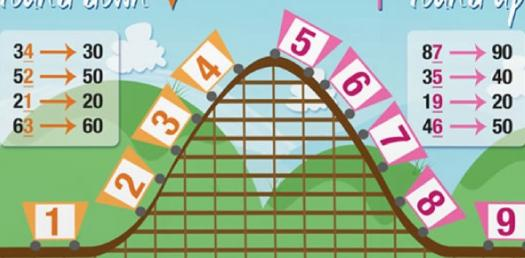# How Well Do You Know Place Value And Rounding?

Approved & Edited by ProProfs Editorial Team
The editorial team at ProProfs Quizzes consists of a select group of subject experts, trivia writers, and quiz masters who have authored over 10,000 quizzes taken by more than 100 million users. This team includes our in-house seasoned quiz moderators and subject matter experts. Our editorial experts, spread across the world, are rigorously trained using our comprehensive guidelines to ensure that you receive the highest quality quizzes.
| By Jaksiboy
J
Jaksiboy
Community Contributor
Quizzes Created: 919 | Total Attempts: 12,132,600
Questions: 10 | Attempts: 196SettingsPlace value in mathematics is the value represented by a number, in decimal system with digits from 0 -9 based on base is the first column left of the decimal place in the unit column. Take a quiz and test your knowledge on place value numbering position.

• 1.

### In the decimal number system, the value of a digit depends on?

• A.

Notation

• B.

Place

• C.

Position

• D.

B and C only

D. B and C only
Explanation
In the decimal number system, the value of a digit depends on the place and position it occupies. The place refers to the position of the digit from the rightmost side, and each place has a value that is a power of 10. The position refers to the specific location of the digit within the number. Therefore, both the place and position determine the value of a digit in the decimal number system.

Rate this question:

• 2.

### Each number have a value of 10 times the place to its?

• A.

Middle

• B.

Right

• C.

Top

• D.

Left

B. Right
Explanation
The correct answer is Right. Each number has a value of 10 times the place to its right. Since the question asks for the number that has a value of 10 times the place to its right, the answer would be the number on the right.

Rate this question:

• 3.

### A number in standard form is separated into groups?

• A.

Tens

• B.

One

• C.

Twos

• D.

Threes

D. Threes
Explanation
In the given question, the groups into which the number in standard form is separated are mentioned as Tens, Ones, Twos, and Threes. The correct answer, "Threes," indicates that the number is separated into groups of three.

Rate this question:

• 4.

### Numbers are broken into group using commas, each of these group is called?

• A.

Float

• B.

Decimal

• C.

Notation

• D.

Period

D. Period
Explanation
Numbers are broken into groups using commas, and each of these groups is called a period. This is a common practice in many countries to make large numbers easier to read and understand.

Rate this question:

• 5.

### 134.9 rounded to tens will give us?

• A.

135.1

• B.

134.9

• C.

130

• D.

135

C. 130
Explanation
When rounding a number to the nearest ten, we look at the digit in the ones place. If it is 0, 1, 2, 3, or 4, we round down to the previous ten. If it is 5, 6, 7, 8, or 9, we round up to the next ten. In this case, the digit in the ones place is 9, which is greater than 5, so we round up. Therefore, 134.9 rounded to tens will give us 140. However, none of the given options is 140. Therefore, the correct answer is 130, which is the previous ten.

Rate this question:

• 6.

### The number 4.56 can be rounded up to?

• A.

4.65

• B.

4.6

• C.

5

• D.

4.55

B. 4.6
Explanation
The number 4.56 can be rounded up to 4.6 because the digit to the right of the desired decimal place is 5 or greater. In rounding, if the digit to the right is 5 or greater, the digit to the left is increased by 1. Therefore, 4.56 is rounded up to 4.6.

Rate this question:

• 7.

### Round 74 to the nearest 10, what do you get?

• A.

7010

• B.

70

• C.

71

• D.

75

B. 70
Explanation
When rounding 74 to the nearest 10, we look at the digit in the ones place, which is 4. Since 4 is less than 5, we round down. Therefore, the number 74 rounds to 70.

Rate this question:

• 8.

### Round 86 to the nearest 10, what do you get?

• A.

87

• B.

88

• C.

90

• D.

80

C. 90
Explanation
When rounding 86 to the nearest 10, we look at the digit in the ones place, which is 6. Since 6 is greater than 5, we round up. Therefore, the number is rounded to the next multiple of 10, which is 90.

Rate this question:

• 9.

### Round 55 to the nearest 10, what do you get?

• A.

61

• B.

60

• C.

50

• D.

65

B. 60
Explanation
To round 55 to the nearest 10, we look at the digit in the ones place, which is 5. Since 5 is greater than or equal to 5, we round up. Therefore, the number 55 rounds up to 60, which is the nearest multiple of 10.

Rate this question:

• 10.

• A.

3.5

• B.

3.14

• C.

3.1

• D.

3.12